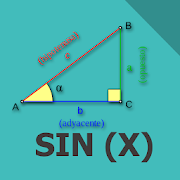# sine, cosine, tangent of an angle - Lumos Educational App Store4.11
Price -fREE
\$0

#### DESCRIPTION:

Trigonometric ratios, fast app to find: sine, cosine, tangent, cotangent, secant and consecante of an angle

#### OVERVIEW:

Sine, cosine, tangent of an angle is a free educational mobile app By .It helps students in grades HS practice the following standards HSF.TF.A.3.

This page not only allows students and teachers download Sine, cosine, tangent of an angle but also find engaging Sample Questions, Videos, Pins, Worksheets, Books related to the following topics.

1. HSF.TF.A.3 : Use special triangles to determine geometrically the values of sine, cosine, tangent for π/3, π/4 and π/6, and use the unit circle to express the values of sine, cosine, and tangent for x, π + x, and 2π - x in terms of their values for x, where x is any real number..

HS

#### STANDARDS:

HSF.TF.A.3

Developer:

Software Version: 1.1

Category:

### RELATED APPSEdSearch WebSearch